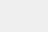# PyTorch 中使用深度学习（CNN和LSTM）的自动图像描述## 介绍• 深度学习基础 – 从人工神经网络开始

• 卷积神经网络（CNNs）的体系结构揭秘

• 教程：使用Keras优化神经网络（带图像识别案例研究）

• 深度学习要点 – Attention的序列到序列建模（使用python）

• 图像描述问题需要什么？

• 解决任务的方法论

• 实施演练

• 下一步？

## 图像描述问题需要什么## 解决任务的方法论Label – [ <start>, A,  man,  and,  a, girl,  sit,  on,  the,  ground,  and,  eat,  . ]
Target – [ A, man,  and,  a,  girl,  sit,  on,  the,  ground,  and,  eat,  ., <end> ]## 实施演练`git clone https://github.com/pdollar/coco.gitcd coco/PythonAPI/makepython setup.py buildpython setup.py installcd ../../git clone https://github.com/yunjey/pytorch-tutorial.gitcd pytorch-tutorial/tutorials/03-advanced/image_captioning/pip install -r requirements.txt`

`chmod +x download.sh./download.sh`

`# Search for all the possible words in the dataset and  # build a vocabulary listpython build_vocab.py     # resize all the images to bring them to shape 224x224python resize.py`

`python train.py --num_epochs 10 --learning_rate 0.01`

`import torchimport torch.nn as nnimport torchvision.models as modelsfrom torch.nn.utils.rnn import pack_padded_sequencefrom torch.autograd import Variableclass EncoderCNN(nn.Module):    def __init__(self, embed_size):        """Load the pretrained ResNet-152 and replace top fc layer."""        super(EncoderCNN, self).__init__()        resnet = models.resnet152(pretrained=True)        modules = list(resnet.children())[:-1]      # delete the last fc layer.        self.resnet = nn.Sequential(*modules)        self.linear = nn.Linear(resnet.fc.in_features, embed_size)        self.bn = nn.BatchNorm1d(embed_size, momentum=0.01)        self.init_weights()            def init_weights(self):        """Initialize the weights."""        self.linear.weight.data.normal_(0.0, 0.02)        self.linear.bias.data.fill_(0)            def forward(self, images):        """Extract the image feature vectors."""        features = self.resnet(images)        features = Variable(features.data)        features = features.view(features.size(0), -1)        features = self.bn(self.linear(features))        return features        class DecoderRNN(nn.Module):    def __init__(self, embed_size, hidden_size, vocab_size, num_layers):        """Set the hyper-parameters and build the layers."""        super(DecoderRNN, self).__init__()        self.embed = nn.Embedding(vocab_size, embed_size)        self.lstm = nn.LSTM(embed_size, hidden_size, num_layers, batch_first=True)        self.linear = nn.Linear(hidden_size, vocab_size)        self.init_weights()        def init_weights(self):        """Initialize weights."""        self.embed.weight.data.uniform_(-0.1, 0.1)        self.linear.weight.data.uniform_(-0.1, 0.1)        self.linear.bias.data.fill_(0)            def forward(self, features, captions, lengths):        """Decode image feature vectors and generates captions."""        embeddings = self.embed(captions)        embeddings = torch.cat((features.unsqueeze(1), embeddings), 1)        packed = pack_padded_sequence(embeddings, lengths, batch_first=True)         hiddens, _ = self.lstm(packed)        outputs = self.linear(hiddens)        return outputs        def sample(self, features, states=None):        """Samples captions for given image features (Greedy search)."""        sampled_ids = []        inputs = features.unsqueeze(1)        for i in range(20):                                    # maximum sampling length            hiddens, states = self.lstm(inputs, states)        # (batch_size, 1, hidden_size),             outputs = self.linear(hiddens.squeeze(1))          # (batch_size, vocab_size)            predicted = outputs.max(1)            sampled_ids.append(predicted)            inputs = self.embed(predicted)            inputs = inputs.unsqueeze(1)                       # (batch_size, 1, embed_size)        sampled_ids = torch.cat(sampled_ids, 1)                # (batch_size, 20)        return sampled_ids.squeeze()`

`python sample.py --image='png/example.png'`<start> 一群长颈鹿站在草地上。<end>

## 然后去哪儿？

• 添加更多的数据 – 当然，这是深度学习模型的通常趋向。您为模型提供的数据越多，表现就越好。您可以将此资源用于其他图像描述数据集 – http://www.cs.toronto.edu/~fidler/slides/2017/CSC2539/Kaustav_slides.pdf

• 使用Attention模型 – 正如我们在本文中所看到的 (深度学习要点 – 使用Attention进行序列建模)，使用注意力模型可以帮助我们微调模型性能。

• 继续研究更大更好的技术 – 研究人员正在研究一些技术 – 例如 使用 强化学习来构建端到端深度学习系统，或者使用新颖的视觉哨兵注意模型。

## 结尾说明点击下方 |  | 了解更多